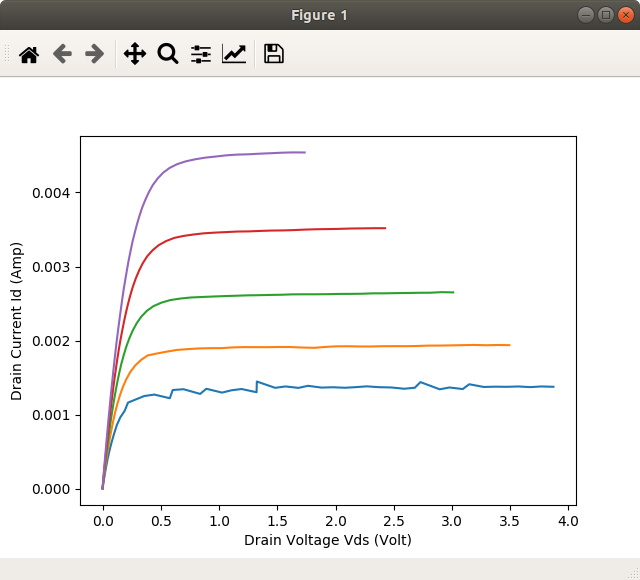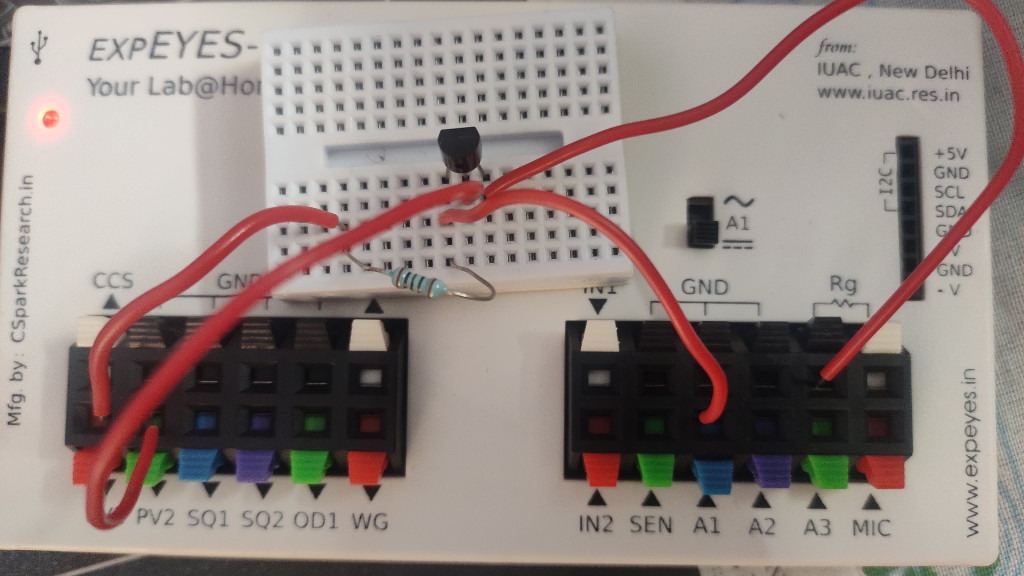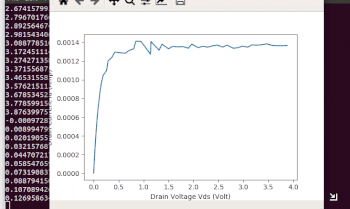# MOSFET Characteristics

## Drain Current Characteristics of a 2N7000 MOSFETBrief Summary
PV2 is connected to the GATE. PV1 is connected to source via a 1K resistor. And DS current is measured as a function of the voltage .

## Introduction

To study n type eMOSFET characteristics.

TODO: Add more details to blog.

### Procedure

• Connect PV2 to GATE.
• Connect PV1 to DRAIN via a 1K resistor
• Source is grounded.
• Connect A1 to the DRAIN. This is the Drain voltage. This enables measuring current as well ( I_DS = (PV1 - A1)/1K )## ResultsThe drain current is plotted against the drain-source voltage for various values of GATE voltage ranging from 1.65 volts to 2.0 Volts.

Below 1.65V gate input, the MOSFET does not conduct enough to obtain characteristics

## Code

``````import eyes17.eyes
p = eyes17.eyes.open()
from pylab import *
import time
#set the inital value of vss
xlabel('Drain Voltage Vds (Volt)')
ylabel('Drain Current Id (Amp)')

pv2 = 1.65
ion() #Enable interactive plotting
while pv2 <= 2.0:
vdsa = []
idra = []
p.set_pv2(pv2)
p.set_pv1(0)
time.sleep(0.2)
#set the intial value of vdd
pv1 = 0.0
while pv1 <= 4.8:
p.set_pv1(pv1)
#Measure voltage vd to find current id and voltage vds
a1= p.get_voltage('A1')
#Find current id for rd=1000 Ohm
idr = (pv1-a1)/1000.
#find vds
vds = a1
#append id & vds in the arrays
idra.append(idr)
vdsa.append(vds)
print(a1,vds, idr)
pv1 += 0.1
plot(vdsa,idra)
pause(0.5)
pv2+=0.05
show()

``````